# Physical Principles

Physical Principles

Movement of teeth in orthodontic treatment requires application of forces and periodontal tissue response to these forces. Force mechanics are governed by physical principles, such as the laws of Newton and Hooke. This chapter presents the basic definitions, concepts, and applicable mechanical principles of tooth movement, laying the groundwork for subsequent chapters.

Newton’s Laws

Isaac Newton’s (1642–1727) three laws of motion, which analyze the relations between the effective forces on objects and their movements, are all applicable to clinical orthodontics.

The law of inertia

The law of inertia analyzes the static balance of objects. Every body in a state of rest or uniform motion in a straight line will continue in the same state unless it is compelled to change by the forces applied to it.

The law of acceleration

The law of acceleration states that the change in motion is proportional to the motive force that is applied. Acceleration occurs in the direction of the straight line in which the force is applied: a = F/m, where a = acceleration, F = force, and m = mass.

The law of action and reaction

The reaction of two objects toward each other is always equal and in an opposite direction. Therefore, to every action there is always an equal and opposite reaction.

Vectors

When any two points in space are joined, a line of action is created between these points. When there is movement from one of these points toward the other, a direction is defined. The magnitude of this force is called a vector, it is shown by the length of an arrow, and its point of application is shown with a point. For example, in Fig 1-1, the line of action of the force vector, which is applied by the labial arch of a removable appliance on the labial surface of the crown of the incisor, is horizontal. The direction is backward (ie, from anterior to posterior), and its amount is signified by the length of the arrow.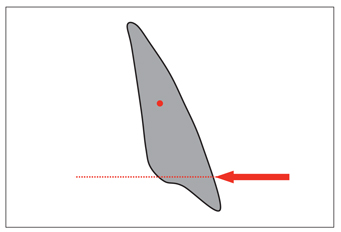Fig 1-1 Force is a vector. The force applied to the incisor is signified by the length of the arrow, and the point of application is on the crown. Its line of action is horizontal, and its direction is from anterior to posterior.

Vectors are defined in a coordinate system. The use of two coordinate axes can be sufficient for vectors on the same plane.

In Fig 1-2a, the resultant (R) of the vectors of different forces (x and y), which are on the same line of action and in the same direction, equals the algebraic sum of these two vectors (x + y). The resultant of two vectors on the same line of action but in opposite directions can be calculated as (x + [–y]) (Fig 1-2b).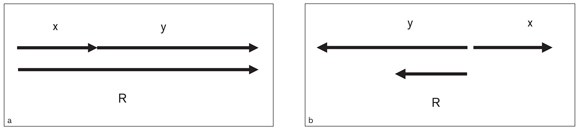Fig 1-2 The resultant (R) of forces (x and y) on the same line of action and direction is R = x + y (a) and the same line of action but in different directions is R = x + (–y) (b).

The resultant of two vectors that have a common point of origin is the diagonal of a parallelogram whose sides are the two vectors (Fig 1-3a). The resultant of the same vectors can also be obtained by joining the tip of a vector parallel to vector y drawn from the tip of vector x to the point of origin of vector x (Fig 1-3b).Fig 1-3 (a) The resultant (R) of the x and y vectors that have the same point of origin is the diagonal of the parallelogram with these vectors used as the sides. (b) R can also be obtained by drawing a vector parallel to vector y and extending from the tip of vector x, then drawing a line joining its tip to the origin of vector x.

Sum of multiple vectors

The sum of multiple vectors is calculated in the same system as the calculation of two vectors. Therefore, the third vector is added to the resultant of the first two vectors, and so on (Fig 1-4).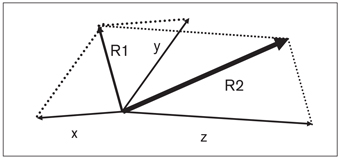Fig 1-4 To find the sum of multiple vectors having the same point of origin, first draw the resultant (R1) of vectors x and y, thus defining the resultant (R2) of the z and R1 vectors (ie, x + y = R1; z + R1 = R2).

Subtraction of two vectors

To define the difference between two vectors, a new vector (–y) is drawn in the opposite direction from the tip of vector x and parallel to vector y, and the point of origin of vector x is joined to the tip of vector –y (Fig 1-5). Thus, the resultant (R) is from the point of origin of vectors x and y toward the tip of vector –y.Fig 1-5 The difference between x and y vectors having the same point of origin can be obtained by drawing a vector (–y) starting from the tip of vector x that runs parallel to the y vector but in the opposite direction; then the tip of vector –y is joined to the point of origin of vectors x and y.

Separating a vector into components

To separate a resultant vector (R) into components, two parallel lines are drawn from the point of origin of that vector toward the components that are searched. By drawing parallels from the R vector’s tip toward these lines, a parallelogram is obtained. The sum of the two components obtained by this method is exactly equal to vector R.

The separation of a resultant vector into components is generally (at the elementary level) realized on x and y reference axes for ease of presentation and trigonometric calculations (Fig 1-6). In fact, for complicated calculations, vectors can be separated into unnumbered directions. Therefore, the x-axis is generally accepted as the horizontal axis, and the y-axis is accepted as the vertical axis. Thus, the component x of vector R can be defined as horizontal and the component y as vertical.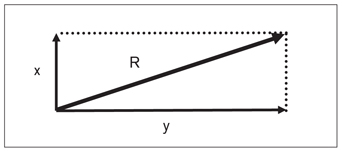Fig 1-6 The separation of a resultant vector into components on an x– and y-axis coordinate system.

Force

Force is the effect that causes an object in space to change its place or its shape. In orthodontics, the force is measured in grams, ounces, or Newtons. Force is a vector having the characteristics of line of action, direction, magnitude, and point of application. In the application of orthodontic forces, some factors such as distribution and duration are also important. During tipping of a tooth, force is concentrated at the alveolar crest on one side and at the apex on the other (Fig 1-7a). During translation, however, the force is evenly distributed onto the bone and root surfaces (Fig 1-7b).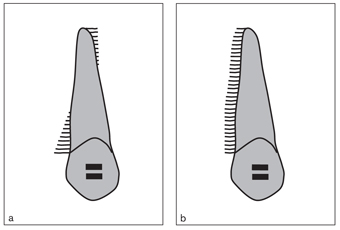Fig 1-7 Distribution of force on the bone and root surfaces in tipping (a) and translatory (b) movements. During tipping, the possibility of indirect bone resorption is high because the forces are concentrated in small areas. Therefore, the forces must be kept as low as possible.

Forces according to their duration

Constancy of force

Clinically, optimal force is the amount of force resulting in the fastest tooth movement without damage to the periodontal tissues or discomfort to the patient. To achieve an optimum biologic response in the periodontal tissues, light, continuous force is important.1 Figure 1-8 compares the amount of loss of force occurring over time on the force levels of two coil springs of high and low load/deflection rates.2

Continuous forces A continuous force can be obtained by using wires with low load/deflection rate and high working range. In the leveling phase, where there is considerable variation in level between teeth, it is advantageous to use these wires to control anchorage and maintain longer intervals between appointments. Continuous force depreciates slowly, but it never diminishes to zero within two activation periods (clinically, this period is usually 1 month); thus, constant and controlled tooth movement results3 (Fig 1-9a). For example, the force applied by nickel titanium (NiTi) open coil springs is a continuous force.

Interrupted forces Interrupted forces are reduced to zero shortly after they have been applied. If the initial force is relatively light, the tooth will move a small amount by direct resorption and then will remain in that position until the appliance is reactivated. After the application of interrupted forces, the surrounding tissues undergo a repair process until the second activation takes place3 (Fig 1-9b). The best example of an active element that applies interrupted force is the rapid expansion screw.

Intermittent forces During intermittent force application, the force is reduced to zero when the patient removes the appliance3 (Fig 1-9c). When it is placed back into the mouth, it continues from its previous level, reducing slowly. Intermittent forces are applied by extraoral appliances.3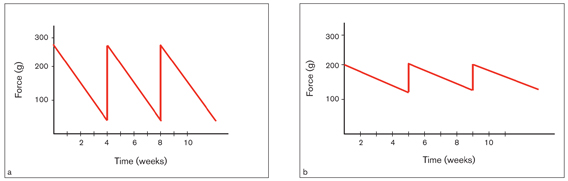Fig 1-8 The loss of force, with time, in springs with high (a) and low (b) load/deflection rates. In the same period (4 weeks), the loss of force in the spring with a high load/deflection rate was approximately 225 g, compared to only 75 g in the spring with a low load/deflection rate. (Reprinted from Gjessing2 with permission.)Fig 1-9 The effects of continuous (a), interrupted (b), and intermittent (c) forces on the periodontal tissues. (Reprinted from Proffit3 with permission.)

Center of Resistance

The point where the line of action of the resultant force vector intersects the long axis of the tooth, causing translation of the tooth, is defined as the center of resistance. Theoretically, the center of resistance of a tooth is located on its root, but the location has been extensively investigated. Studies show that the center of resistance of single-rooted teeth is on the long axis of the root, approximately 24% to 35% of the distance from the alveolar crest.410

The center of resistance is sometimes confused with the center of mass. The center of mass is a balance point of a free object in space under the effect of gravity. A tooth, however, is a restrained object within the periodontal and bony structures surrounded by muscle forces. Therefore, the center of resistance must be considered a balance point of restrained objects.

The center of resistance is unique for every tooth; the location of this point depends on the number of roots, the level of the alveolar bone crest, and the length and morphology of the roots. Therefore, the center of resistance sometimes changes with root resorption or loss of alveolar support because of periodontal disease (Fig 1-10). For example, in the case of loss of alveolar support, this point moves apically.11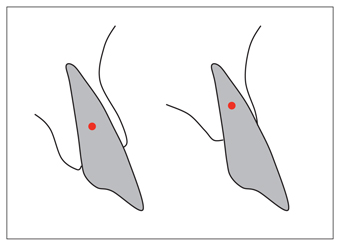Fig 1-10 The in response to a loss of alveolar bone or periodontal attachment.

Center of Rotation

The center of rotation is the point around which the tooth rotates. The location of this point is dependent on the force system applied to the tooth, that is, the moment-to-force (M/F) ratio. When a couple of force is applied on the tooth, this point is superimposed on the center of resistance (ie, the tooth rotates around its center of resistance). In translation it becomes infinite,meaning there is no rotation. This subject is explained in greater detail in the M/F ratio section later in the chapter.

Moment

Moment is the tendency for a force to produce rotation or tipping of a tooth. It is determined by multiplying the magnitude of the force (F) by the perpendicular distance (d) from the center of resistance to the line of action of this force: M = F × d (Fig 1-11). In orthodontic practice, it is usually measured in gram-millimeters, or g-mm, which means grams × millimeters. Forces passing through the center of resistance do not produce a moment, because the distance to the center of resistance is zero. Hence, the tooth does not rotate; it translates (Fig 1-12). Because the moment depends on the magnitude of the force and the perpendicular distance to the center of resistance, it is possible to obtain the same rotational effect by doubling the distance and reducing the magnitude of the force by half, or vice versa. Even when the force is not excessive but the distance from the center of resistance to the line of action is significant, the periodontal tissues may be adversely affected because of the large moment.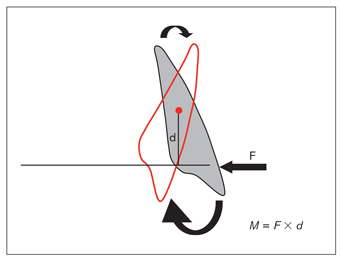Fig 1-11 The line of action of any force (F) not passing through the center of resistance creates a moment (M), which is a rotational or tipping effect on the tooth. According to the formula M = F × d, a moment is proportional to the magnitude of force and the distance (d) perpendicular from its line of action to the center of resistance.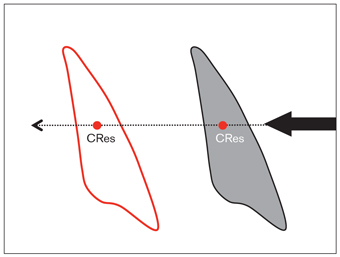Fig 1-12 A force having a line of action passing through the center of resistance (CRes) causes translation of the tooth. During this movement, the center of resistance moves along the line of action of the force.

Couple